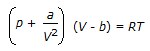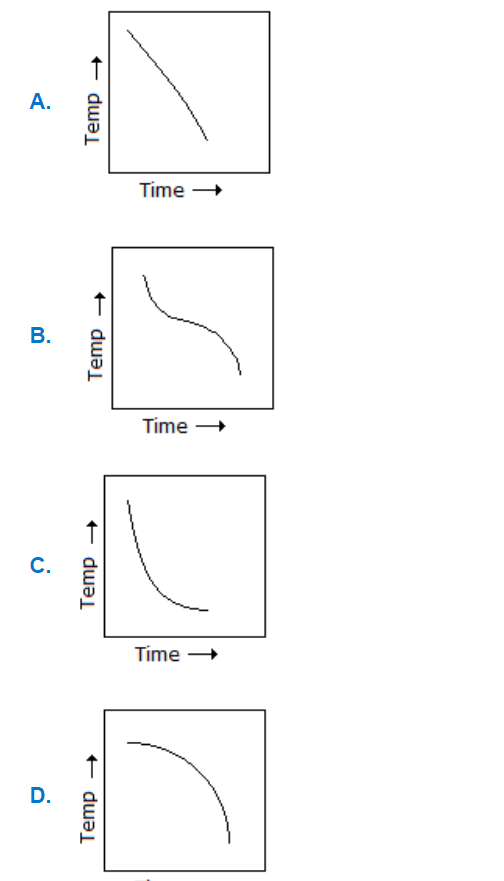# E-PolyLearning

 6. Internal energy of a gas obeying Vanderwall's equation of state given below, depends upon itsa. pressure & temperature. b. pressure & specific volume. c. temperature & specific volume. d. temperature only.

 7. Which of the following represents the correct time-temperature curve when a block of metal is heated above room temperature & then left to cool in the room following Newton's law of cooling in which the rate of temperature drop will go on decreasing with the passage of time.a. A b. B c. C d. D